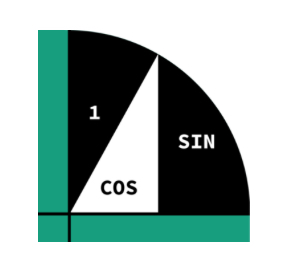# Calculus notes

RadMaths home page $\text{Note created in February,2021}$ This note will be updated once in a while :)
Practice questions are being uploaded :) You will find them in nested buttons :) Chapters with trailing ‘+test‘s have questions :)
ALL QUOTES ARE COPIED FROM BRILLIANT WIKI WITHOUT CHANGE.

# Calculus with only one variable—$y=f(x)$

Chapters:
1.Limits
2.Differentiation and derivatives
3.Integrals
4.Infinite sums

## 1. Limitsoff we go!

## 2. Differentiation and derivativesoff again!

## 3. IntegralsGabriel’s horn(trumpet): TOOT!

## 4. Infinite sumsWhooo!Note by Jeff Giff
6 months ago

This discussion board is a place to discuss our Daily Challenges and the math and science related to those challenges. Explanations are more than just a solution — they should explain the steps and thinking strategies that you used to obtain the solution. Comments should further the discussion of math and science.

When posting on Brilliant:

• Use the emojis to react to an explanation, whether you're congratulating a job well done , or just really confused .
• Ask specific questions about the challenge or the steps in somebody's explanation. Well-posed questions can add a lot to the discussion, but posting "I don't understand!" doesn't help anyone.
• Try to contribute something new to the discussion, whether it is an extension, generalization or other idea related to the challenge.

MarkdownAppears as
*italics* or _italics_ italics
**bold** or __bold__ bold
- bulleted- list
• bulleted
• list
1. numbered2. list
1. numbered
2. list
Note: you must add a full line of space before and after lists for them to show up correctly
paragraph 1paragraph 2

paragraph 1

paragraph 2

[example link](https://brilliant.org)example link
> This is a quote
This is a quote
    # I indented these lines
# 4 spaces, and now they show
# up as a code block.

print "hello world"
# I indented these lines
# 4 spaces, and now they show
# up as a code block.

print "hello world"
MathAppears as
Remember to wrap math in $$ ... $$ or $ ... $ to ensure proper formatting.
2 \times 3 $2 \times 3$
2^{34} $2^{34}$
a_{i-1} $a_{i-1}$
\frac{2}{3} $\frac{2}{3}$
\sqrt{2} $\sqrt{2}$
\sum_{i=1}^3 $\sum_{i=1}^3$
\sin \theta $\sin \theta$
\boxed{123} $\boxed{123}$

Sort by:

add this to your chapter 4: $\frac{d}{dx}\big( f(g(x))\big) \equiv f'(g(x))g'(x)$

- 6 months ago

Yeah I was working on that bit :)

- 6 months ago

# If You Have A Problem Reply Below This Comment To Reduce Space

- 5 months ago

Post problems HERE! :)

- 5 months ago

question 1d of part 1-4 (complicated derivation) has an incorrect answer, the correct answer would be $\left(\frac{\cos x+e^{\sin x}\cdot\cos x}{2\sqrt{\left(\sin x+e^{\sin x}\right)}}\right)$

- 5 months ago

Thank you very much for telling me! But why can’t you guys just reply the comment saying ‘post problems below to reduce space’? It will be terribly difficult to sort through stuff later on :/

- 5 months ago

Awesome notes! Would you be open to converting them onto memorize.ai so people can use them as flash cards?

- 5 months ago

Nope :) by the way next time you have a problem reply below my titiled comment to reduce space :)

- 5 months ago